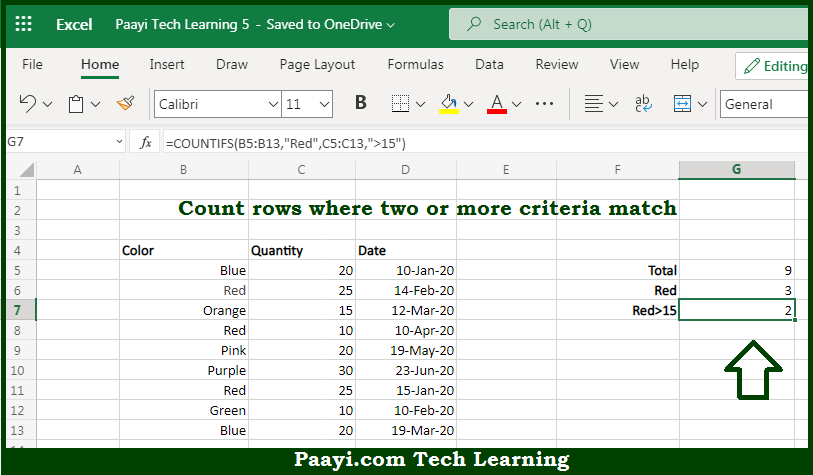# Learn How to Count if Two Criteria Match in Microsoft Excel

Written by | 0 Comments | 452 Views

In this article, you will learn how to COUNT various things in Microsoft Excel using a single or combination of functions and its purpose in Microsoft Excel. You will also get to know how to Count if Two Criteria Match and see the generic formula.

Count if Two Criteria Match in Microsoft Excel

The main purpose of this formula is to count rows if the two criteria match. Here we will learn how to count rows where two or more criteria match in Microsoft Excel. That implies, with the help of a formula based on the COUNTIFS function you can able to count rows where two or more criteria match. So, with the help of this formula, you can able to count rows where two (or more) criteria match.

General Formula to Count if Two Criteria Match

=COUNTIFS(range1,critera1,range2,critera2)

The Explanation for the Count if Two Criteria MatchSo we know that with the help of the given formula above you can able to count rows if the two criteria match. Here we will learn how to count rows where two or more criteria match in Microsoft Excel. As we know that, the COUNTIFS function takes multiple criteria in pairs, which means each pair contains one range and the associated criteria for that range. To give the result of a count, all conditions must match. It should be noted that to add more conditions, just add another range/criteria pair. So, with the help of this formula, you can able to count rows if the two criteria match.